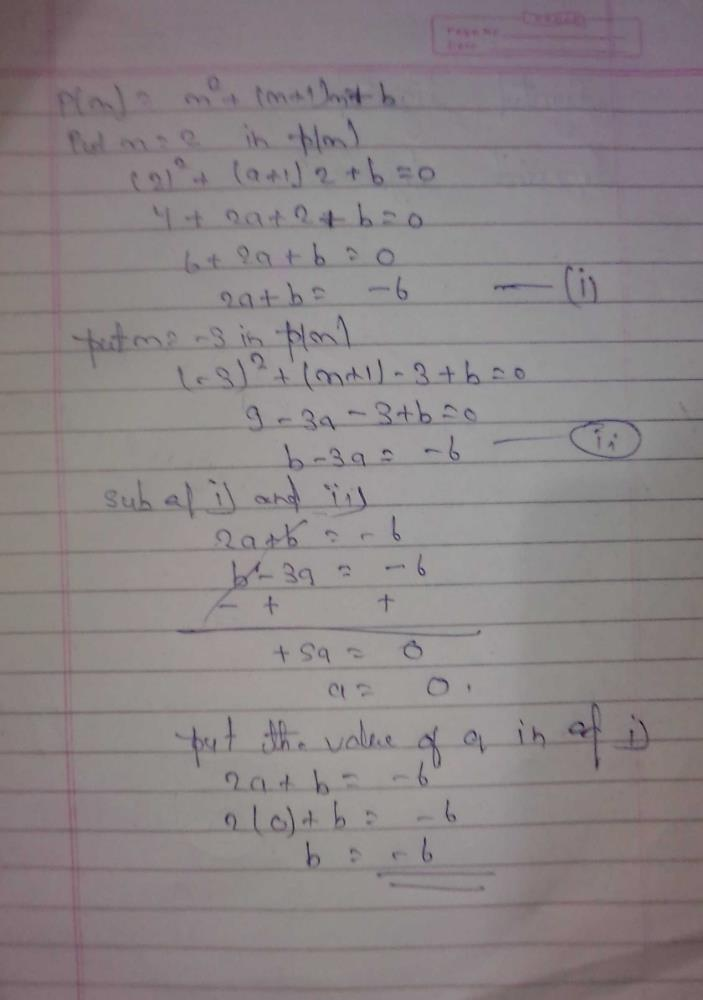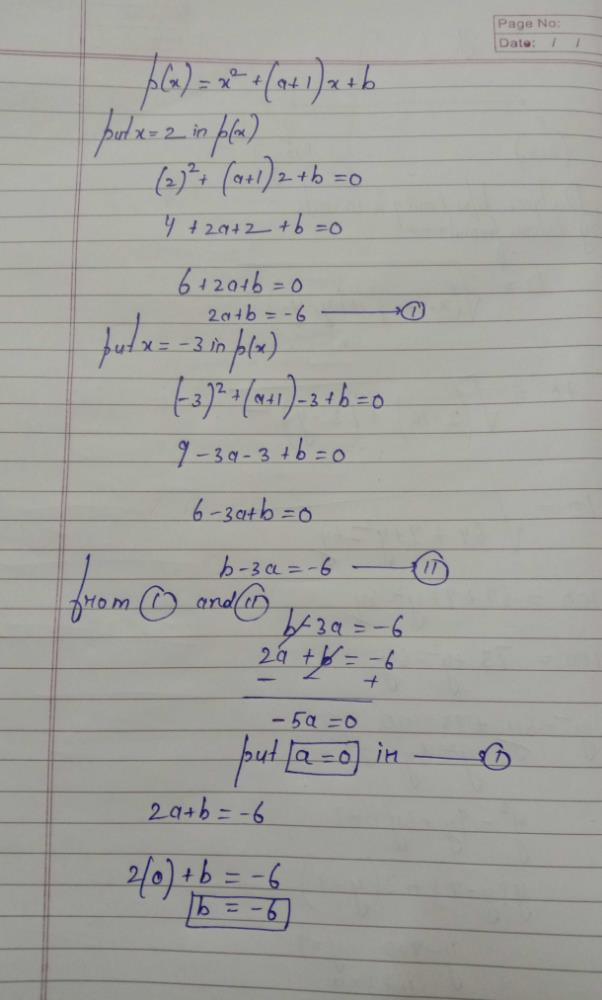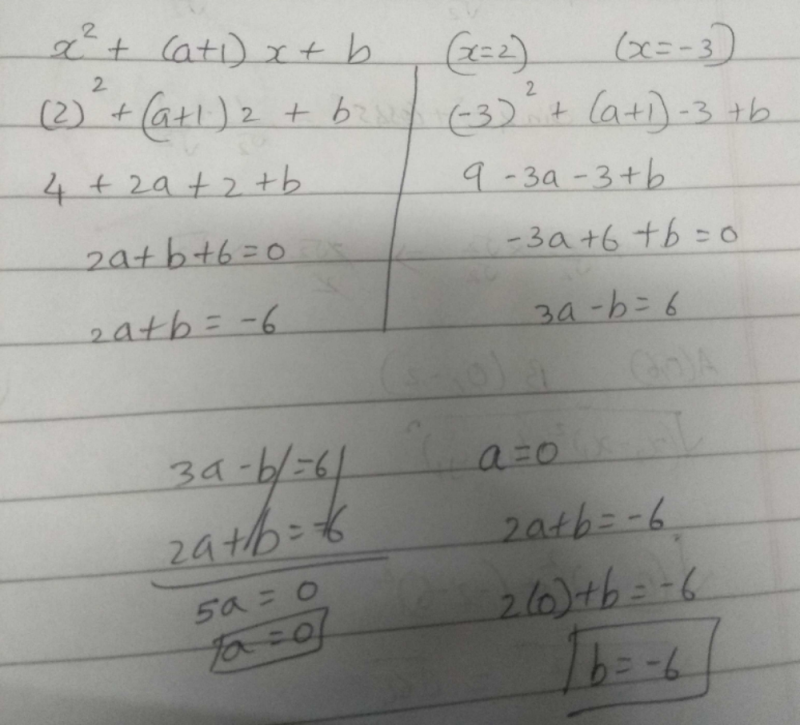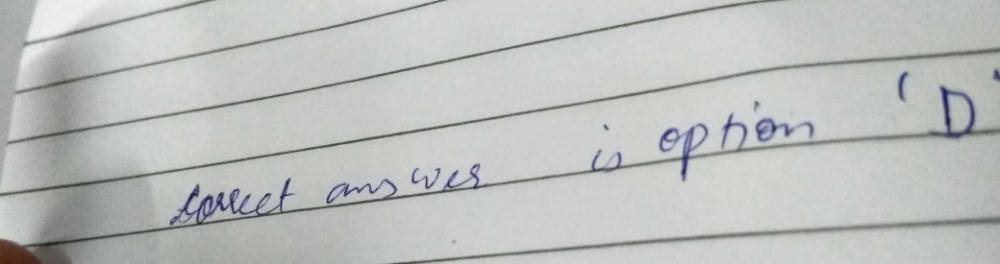Courses

# If the zeros of the quadratic polynomial x2 + (a + 1)x + b are 2 and -3, thena)a = - 7, b = -1b)a = 5, b = - 1c)a = 2, b =- 6,d)a =0, b = - 6Correct answer is option 'D'. Can you explain this answer? Related Test: MCQ : Polynomials - 1

## Class 10 Question

By Arifa Ashrafi · Mar 28, 2020 ·Class 10
Abhi Prajapati answered Mar 28, 2020Nirmal Kumar answered Feb 04, 2020Gautham Rao answered 2 weeks ago
A is 0 b is -6

Malavika Pmk answered Jan 26, 2020Narinder Sharma answered 2 weeks agoRuhi Sharma answered 2 weeks ago
Answer is D (a =0, b = - 6)

Sp Singh answered 2 weeks ago
D